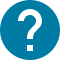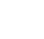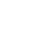学部前期課程
HOME 学部前期課程 全学自由研究ゼミナール(PEAK)(数学I②)(MathematicsI②)

# 全学自由研究ゼミナール(PEAK)(数学I②)(MathematicsI②)

PEAK: Mathematics I (Spring 2022) – Multivariable Calculus
Based on calculus for single variable functions we learned last semester, we continue to study calculus for multivariable functions. In particular, we will extend many of the basic concepts we learned so far to functions of two or more variables. Although many of the ideas that we developed for single variable functions will carry over naturally, there exist intrinsic differences between single and multivariable functions. So sometimes we need new tools and new ideas. In this course we introduce partial derivatives, multiple integrals, and their applications.

コース名

30745
CAS-TC1200S1

S1 S2

2

NO

Lecture 1: Introduction and readiness check brief review on calculus for single variable functions Lecture 2: Functions of several variables examples of multivariable functions, graph of a function of two variables Lecture 3: Partial derivatives definition of of partial derivatives, geometric interpretation of partial derivatives Lecture 4: Tangent plane and chain rule differentiable functions, tangent planes, chain rule Lecture 5: Higher-order partial derivatives definition of higher-order partial derivatives, equality of mixed partials Lecture 6: Taylor's theorem and extreme values Taylor's theorem, Hessian matrix, second derivative test, the least-squares technique Lecture 7: Implicit function theorem implicit function theorem for 1)f(x; y) = c, 2)f(x; y; z) = c, and 3) three variables two equations Lecture 8: Lagrange multiplier constrained optimaztion, the method of Lagrange multipliers Lecture 9: Double integrals double integrals over a rectangle, double integrals over a general domain Lecture 10: Itereated integral calculation of double integrals using iterated integrals, geometric interpretation of double integrals Lecture 11: Change of variables polar coordinate systems, various coordinate systems and Jacobians Lecture 12: Improper integral improper integrals in one variable, improper integral in two variables, Gamma function, Gaussian integral Lecture 13: Line integrals definition of line integrals, vector field, Green's theorem

Lecture notes and homework will be uploaded to ITC-LMS each week.

- Homework (full 20 points): Submit your homework each week. Homework score will be given based on your performance. - Final exam (full 80 points).